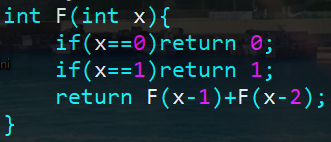## JC2：递推，递归与分治

f=0; f=1;

for(int i=1; i<=n; i++) f[i]=f[i-1]+f[i-2];

f(n)=g(n,f(n-1)) n>0

f(0)=a n=0